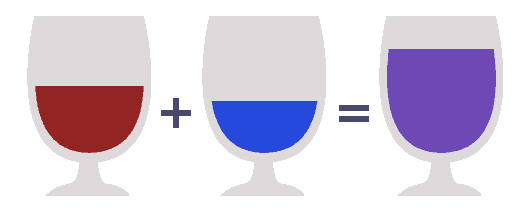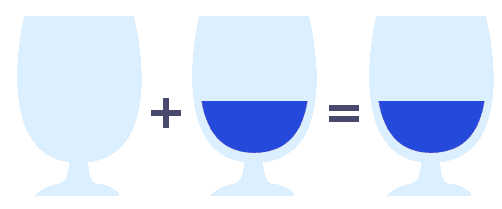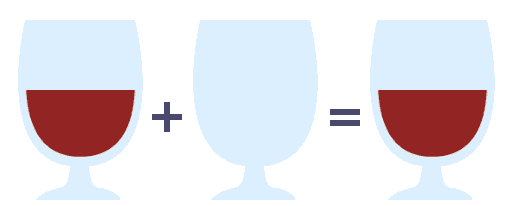# 函数式编程模式：单位元(幺元 Identity Element)

单位元(又称幺元，英文Identity Element)是代数和函数式编程中常见的模式，它实际就是关于空的定义，是不是有点像老子道德经中的“无”“空虚”。``````pour(emptyGlass, x) = x
pour(x, emptyGlass) = x``````

``````add(0, x) = x
add(x, 0) = x``````

``````concat("", x) = x
concat(x, "") = x``````

``````concat([], x) = x
concat(x, []) = x``````

``````x ⊕ e = x
e ⊕ x = x``````

``````min(∞, x) = x;
min(x, ∞) = x;``````

``````mul(1, x) = x;
mul(x, 1) = x;``````

``````f ∘ id = f
id ∘ f = f``````